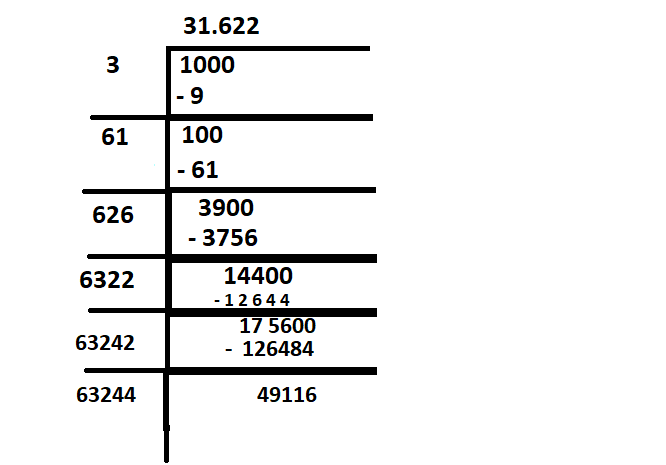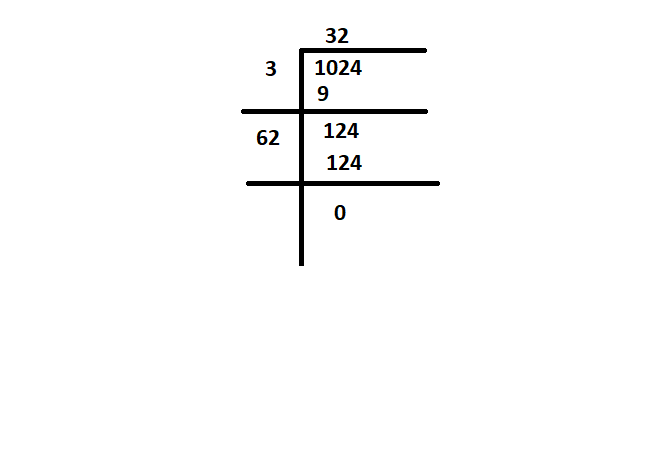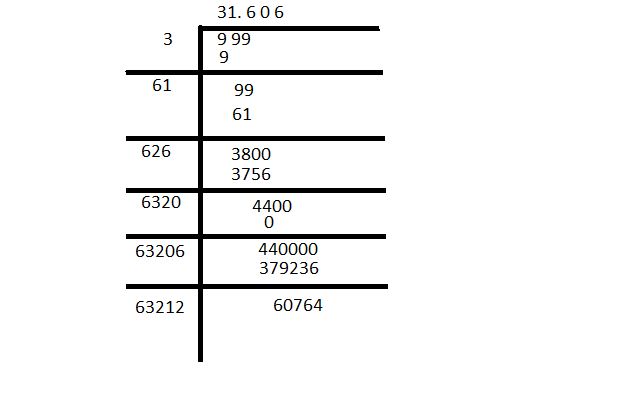GeeksforGeeks App
Open AppBrowser
Continue

# Find the smallest four digit number which is a perfect square

The arithmetic value which is used for representing the quantity and used in making calculations are defined as Numbers. A  symbol like “4,5,6” which represents a number is known as numerals. Without numbers, we can’t do counting of things, date, time, money, etc. these numbers are also used for measurement and used for labeling.

The properties of numbers make them helpful in performing arithmetic operations on them. These numbers can be written in numeric forms and also in words.

For example, 3 is written as three in words, 35 is written as thirty-five in words, etc. Students can write the numbers from 1 to 100 in words to learn more.

There are different types of numbers, which we can learn. They are whole and natural numbers, odd and even numbers, rational and irrational numbers, etc

What is a Number System?

A Number System is a method of showing numbers by writing, which is a mathematical way of representing the numbers of a given set, by using the numbers or symbols in a mathematical manner. The writing system for denoting numbers using digits or symbols in a logical manner is defined as Number System.

We can use the digits from 0 to 9 to form all the numbers. With these digits, anyone can create infinite numbers.

For example, 156,3907, 3456, 1298, 784859, etc

### What is a Square Root?

The value of a number of square roots, which on multiplication by itself gives the original number. Suppose, a is the square root of b, then it is represented as a = √b or we can express the same equation as a2 = b. Here,’√’ this symbol we used to represent the root of numbers is termed as radical. The positive number when it is to be multiplied by itself represents the square of the number. The square root of the square of any positive number gives the original number.

For example, the square of 4 is 16, 42 = 16, and the square root of 16, √16 = 4. Since 4 is a perfect square, hence it is easy to find the square root of such numbers, but for an imperfect square, it’s really tricky.

Square Root is represented as  ‘√’. It is called a radical symbol. To represent a number ‘a’ as a square root using this symbol can be written as: ‘√a‘, where a is the number.

The number here under the radical symbol is called the radicand. For example, the square root of 4 is also represented as a radical of 4. Both represent the same value.

and the formula to find the square root is: b = √a

Properties of Square Roots

It is defined as a one-to-one function that takes a positive number as an input and returns the square root of the given input number.

f(x) = √x

For example, here if x = 9, then the function returns the output value as 3.

These are properties of the square root as follows:

• If a number is a perfect square number, then there definitely exists a perfect square root.
• If a number ends with an even number of zeros (0’s), then we can have a square root.
• The two square root values can be multiplied. For example, √3 can be multiplied by √2, then the result will be √6.
• When two same square roots are multiplied, then the result must be a radical number. It shows that the result is a non-square root number. For example, when √7 is multiplied by √7, the result obtained is 7.
• The square root of negative numbers is undefined. hence the perfect square cannot be negative.
• Some of the numbers end with 2, 3, 7, or 8 (in the unit digit), then the perfect square root does not exist.
• Some of the numbers end with 1, 4, 5, 6, or 9 in the unit digit, then the number will have a square root.

It is easy to find the square root of a number that is a perfect square.

Perfect squares are those positive numbers that can be written as the multiplication of a number by itself. or you can say that a perfect square is a number which is the value of power 2 of any integer.

The number that can be expressed as the product of two equal integers. For example, 16 is a perfect square because it is the product of two equal integers, 4 × 4 = 16. However, 24 is not a perfect square because it cannot be expressed as the product of two equal integers. (8 × 3 = 24).

The number which is obtained by squaring a whole number.

If we assume N is a perfect square of a whole number y, this can be written as N = the product of y and y = y2

So, the perfect square formula can be expressed as:

N = Y2

Let’s Use the formula with values.

If y = 5, and N = y2.

This means, N = 52 = 25.

Here, 25 is a perfect square because it is the square of a whole number.

With the help of square roots, we can identify  whether a number is a perfect square or not and if we calculate the square root of the given number.

If the square root is a whole number then the given number will be a perfect square, and if the square root value is not a whole number, then the given number is not a perfect square.

For instance, to check whether 24 is a perfect square or not, we will calculate its square root. √24 = 4.898979. As we can see, 4.898979 is not a whole number, so, 24 is not a perfect square.

Lets take another example of

The number 49. √49 = 7. We can see that 7 is a whole number, therefore, 49 is a perfect square.

### Find the smallest four-digit number which is a perfect square

The squares of all integers are called perfect square numbers.

The method of finding the smallest four-digit number which is a perfect square is explained below:

As we all know that the smallest 4 digit number is 1000. But its not a perfect square number.and after the square root of 1000 we are clear that the smallest four-digit number which is a perfect square should be near to 1000.

Let us try some numbers which are a perfect square,

So here square root of 1000 is 31.622 and is not a whole number,

31 is the number which has perfect square which has three digit (31) =  961, before four-digit number 1000, 1000 is not a perfect square of number as shown above in image…

So let’s try with the square of 32:

N = (32)2 = 32 × 32 = 1024square root of 1024 is 32

With the square root method of 1024 is 32 which is a perfect square, so 1024 is the smallest four-digit number that has perfect square

### Similar Questions

Question 1: Find the smallest three digit number which has perfect square?

Solution:

Here smallest two digit number we all know is 10

so if we find the square of two consecutive numbers

9 is,  92 = 81

10 is, 102 = 100

and √100 = 10

If the square root is a whole number then the given number will be a perfect square, and if the square root value is not a whole number, then the given number is not a perfect square.

Here it clearly shows that 100 is the least three digit number which has perfect square

Question 2: Find the largest three digit number which has perfect square?

Solution:

Here largest three digit number, we have is 999

So if we find the square root of 999square root of 999

here largest three digit number is 999 but its not a perfect square as square root of 999 is 31.606..

So as we continue to search the number which is closest to the number we have 31.606 is 31 or 32

Here (32)2 = 1024 means 32 is not the correct number to find the largest three digit number which has perfect square…..

Now, we will try another number that is 31

so , (31) 2 =  961

the answer to question to find the largest three digit number which has perfect square is 961 whose square root is 31.

My Personal Notes arrow_drop_up
Related Tutorials